## RS Aggarwal Class 9 Solutions Chapter 2 Polynomials Ex 2C

These Solutions are part of RS Aggarwal Solutions Class 9. Here we have given RS Aggarwal Solutions Class 9 Chapter 2 Polynomials Ex 2C.

Other Exercises

Using remainder theorem, find the remainder when :

Question 1.
Solution:
f(x) = (x3 – 6x2 + 9x + 3)
Let x-1 = 0, then x = 1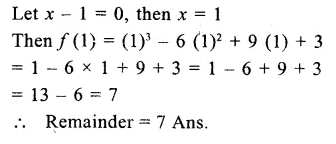Question 2.
Solution:
f(x) = 2x3 – 5x2 + 9x – 8)
Let x-3 = 0, then x = 3Question 3.
Solution:
f(x) = (3x4 – 6x2 – 8x + 2)
Let x-2 = 0, the x = 2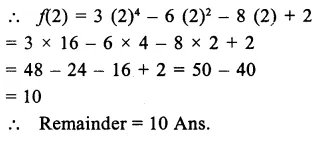Question 4.
Solution:
f(x) = (x3 – 7x2 + 6x + 4)
Let x-6 = 0,then x=6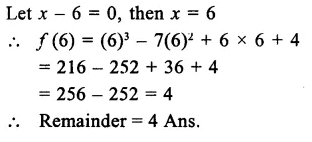Question 5.
Solution:
f(x)=(x3 – 6x2 + 13x + 60)
Let x+2=0,then x=-2Question 6.
Solution:
f(x)=(2x4 + 6x3 + 2x2 + x – 8)
Let x+3=0,then x=-3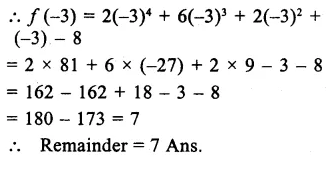Question 7.
Solution:
f(x)=(4x3 – 12x2 + 11x – 5)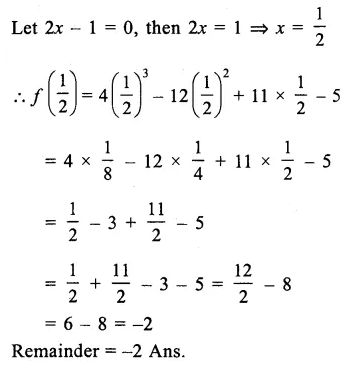Question 8.
Solution:
f(x)=(81x4 + 54x3 – 9x2 – 3x + 2)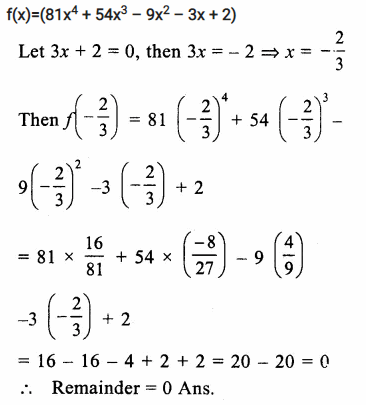Question 9.
Solution:
f(x)=(x3 – ax2 + 2x – a)
let x-a=0, then x=a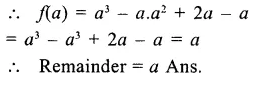Question 10.
Solution:
f(x)=(ax3+ 3x2 – 3)
g(x)=(2x3 -5x + a)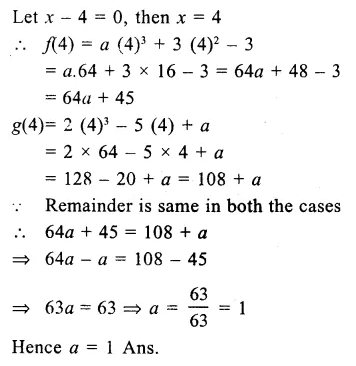Question 11.
Solution:
f(x)=x4 – 2x3 + 3x2 – ax + b
let x-1=0, then x=1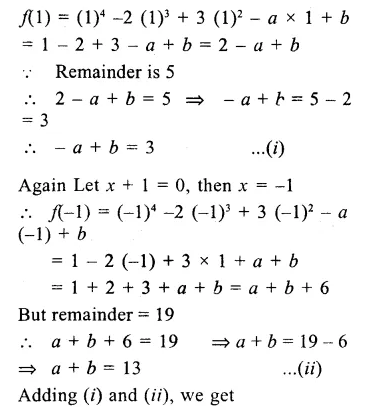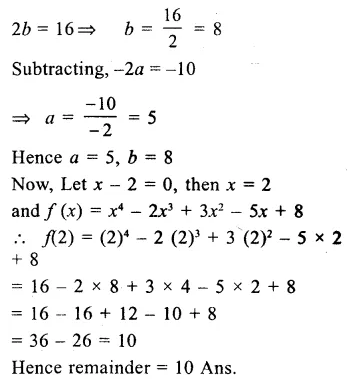Hope given RS Aggarwal Solutions Class 9 Chapter 2 Polynomials Ex 2C are helpful to complete your math homework.

If you have any doubts, please comment below. Learn Insta try to provide online math tutoring for you.